• 主要为大家详细介绍了python广度优先搜索得到两点间最短路径，具有一定的参考价值，感兴趣的小伙伴们可以参考一下
• 这篇文章主要为大家详细介绍了python广度优先搜索得到两点间最短路径，具有一定的参考价值，感兴趣的小伙伴们可以参考一 前言 之前一直写不出来，这周周日花了一下午终于弄懂了， 顺便放博客里，方便以后忘记了再看...
• Python 广度优先搜索（BFS） 广度优先算法Python实现使用到的list方法两个实例最短路径最长路径 广度优先算法 我的理解是在搜索时向一切可能进行的方向搜索，拿经典的走迷宫问题举例子，每次遇到十字路口，就分出...
Python 广度优先搜索（BFS） 广度优先算法Python实现使用到的list方法两个实例最短路径最长路径
广度优先算法
我的理解是在搜索时向一切可能进行的方向搜索，拿经典的走迷宫问题举例子，每次遇到十字路口，就分出三个分身把三个可能的方向都走一遍，这里采用一个数据结构队列，先进先出的保存路径，可以走的状态就保存一下，每次走之前先从队列里面取出来一个状态点，从这一点开始走。
Python实现
Python中已经实现了队列（queue）的结构，直接import就可以使用，然而queue在py2和py3中是有区别的，我在刷oj的时候遇到了这个问题。不过Python给用户提供了比较强大的数据结构列表：list，干脆直接用最基本的list结构来处理这个问题。
使用到的list方法

list.append(obj) 在list尾部添加元素obj
list.pop([index=-1]) 移除并返回列表中的某一项 默认为最后一项 移除第二项可以写作list.pop(1)
del Python的删除关键字，移除第一项可以写作del list

两个实例
最短路径

Pythontip 24一马当先1
下过象棋的人都知道，马只能走’日’字形（包括旋转90°的日），现在想象一
下，给你一个n行m列网格棋盘，
棋盘的左下角有一匹马，请你计算至少需要几步可以将它移动到棋盘的右上
角，若无法走到，则输出-1.
如n=1，m=2,则至少需要1步；若n=1，m=3,则输出-1。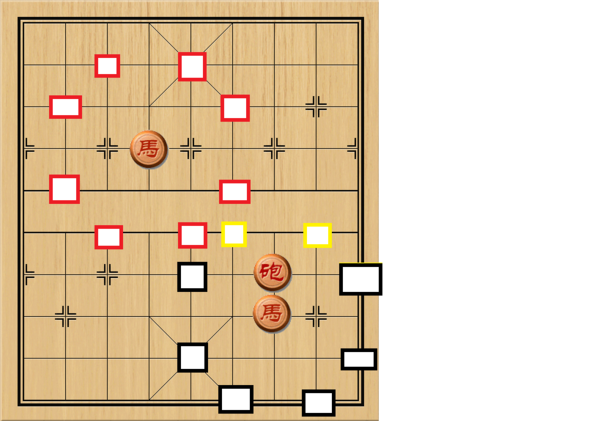这个题可以广度搜索，基本思路是每次向八个方向中没走过的方向都走一遍，可以证明重复走过某一点一定会比只经过这一点一次路径要长，建立一个used数组保存使用过的点，不走重复路。建立一个map数组记录距离。题目中没有设置陷阱蹩马腿，还算比较良心。ac代码如下：
# n = 1
# m = 3

map = [[100000000 for x in range(m + 1)] for y in range(n + 1)]
used = [[0 for x in range(m + 1)] for y in range(n + 1)]
map = 0
dx = [1, -1, 1, -1, 2, -2, 2, -2]
dy = [2, -2, -2, 2, 1, -1, -1, 1]  # 八个扩展方向
horses = []
horses.append([0, 0])
while (horses):
h = horses  # 取出第一项
del horses  # 删除第一项
if h == n and h == m:  # 已达终点
break
for i in range(8):  # 八个方向探索
nx = h + dx[i]
ny = h + dy[i]
if nx >= 0 and nx <= n and ny >= 0 and ny <= m and used[nx][ny] == 0:
map[nx][ny] = map[h][h] + 1  # 记录长度
used[nx][ny] = 1  # 已经使用
horses.append([nx, ny])  # 将该点保存在list中
if map[n][m] != 100000000:
print(map[n][m])
else:
print(-1)

最长路径

Pythontip 78 滑雪比赛2
我们把场地分为一个个的格子，给每个格子标定一个整数，代表这个格子所代表的地面的海拔高度。
比赛的参赛者可以从任意一个格子开始，但只能向相邻的四个格子移动，并且目地格子的高度必须
小于现在所在格子的高度。我们假设从一个格子滑行到另一个格子所用的时间为1个单位时间。
现在告诉你滑雪场的大小为n*m, 并给你一个n行m列的整数二维列表H，表示每个格子的海拔高度。
请你计算出在这个场地上最长能滑行多少时间。
如：
n = 4
m = 4
H= [
[1, 2, 3, 4],
[5,6,7,8],
[9,10,11,12],
[13,14,15,16]
]
则输出 6.

这里显然是从最高点出发，在最低点结束，但是第一个到达最低点的是路径最短的，这里可以换个思路，在list中的点除了x，y坐标外再加上一个新变量t，这个新变量表示了路程或时间，每次都在list中取前端，直到list中没有元素，那么最后一个取出的元素是在list中待的时间最长的，那么它一定表示最长路径。
ac代码如下：
# ~ n = 4
# ~ m = 4
# ~ H= [
# ~ [1, 2, 3, 4],
# ~ [5,6,7,8],
# ~ [9,10,11,12],
# ~ [13,14,15,16]
# ~ ]
maxx = 0
maxy = 0
dx = [0, 1, 0, -1]
dy = [1, 0, -1, 0]  # 四个可能的方向
for i in range(n):  # 寻找最高点
for j in range(m):
if H[i][j] > H[maxx][maxy]:
maxx = i
maxy = j
temp = [maxx, maxy, 0]  # 第一个元素 起点坐标加初始路程
que = []
que.append(temp)
while (que):
temp = que.pop(0)  # 取第一项
for i in range(4):  # 四方向搜索
nx = temp + dx[i]
ny = temp + dy[i]
if n > nx >= 0 and m > ny >= 0 and H[nx][ny] < H[temp][temp]:
t = [nx, ny, temp + 1]  # 新位置的信息
que.append(t)  # 保存在list中
print(temp)  # 输出最后元素的路程


一马当先 ↩︎

滑雪比赛 ↩︎


展开全文算法
• 广度优先搜索的官方概念，请自行搜索查阅，这里仅做剖析帮助理解。 意义 学习算法，首先一定要搞清楚每种算法使用的具体场景，主要解决什么问题的，这是学以致用的前提条件。广度优先搜索主要解决2个问题： ...
一 .相关概念及学习的意义
广度优先搜索的官方概念，请自行搜索查阅，这里仅做剖析帮助理解。

意义

学习算法，首先一定要搞清楚每种算法使用的具体场景，主要解决什么问题的，这是学以致用的前提条件。广度优先搜索主要解决2个问题：

1.起点到终点是否有路径；2.起点到终点最短路径，这点需要简单说明下，所谓最短指的分段最少，而不考虑每段的距离多长，是假设每个分段距离相等而言。当然如果考虑分段距离，那属于另外一种算法，这里先提个概念：迪克斯拉特算法，是一种加权算法。

在实际应用中应用非常广发，比如计算网络跳点，人工智能跳棋设计等。广度优先搜索（Breadth-first search，简称BFS）是基于图这种数据结构的其中一种普通使用的算法。

图（Grapth）

是对树的一种扩展。在计算机科学技术范畴中，图是一种应用非常广泛的数据结构，如人脉关系中一度，二度等人脉，族谱关系等。要了解和使用BFS解决实际问题，就要对图有个初步了解，图分无向图和有向图。图是有一系列的顶点和边组成。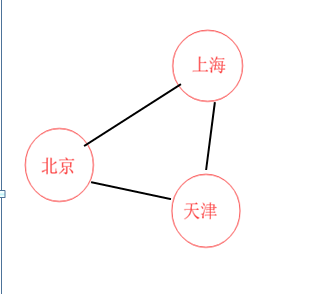图1：无向图：意味着三个城市之间可以互通，如果加了箭头，则为有向图，表明城市之间是无法往返的。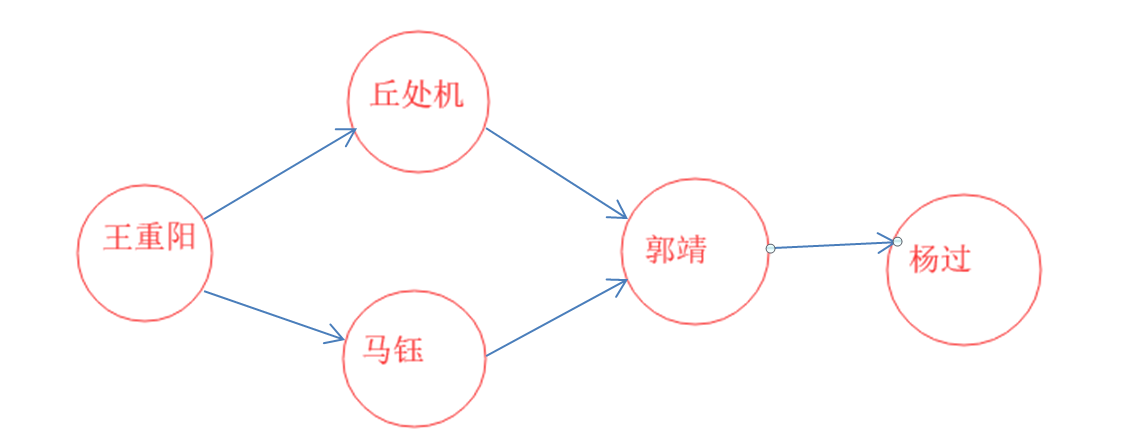图2：有向图，带有箭头，表明师徒关系，如果去掉箭头就乱了辈分了

图的Python实现

对图有个基本了解以后，那么怎么用计算机科学表示呢，看下面这个有向图。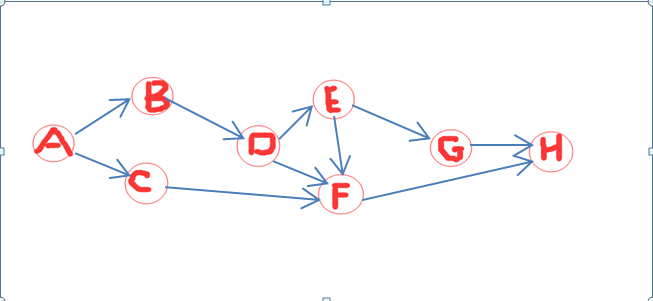图3：有向图

现在我们通过Python的字典Dict类型来表示这个图，这里需要说明下，注意两点：1.要了解BFS,就要对队列有所了解，队列：先进先出，是有序的，广度优先搜索就是解决最短路径问题，当然要知道谁先到达终点；2.为什么要用字典呢，字典是无序的，这里无序是针对顶点的，初始化顶点是没有先后要求的。

代码片段如下：

from collections import deque

def data_ini():
grapth={}
grapth["A"]=["B","C"]
grapth["B"]=["D"]
grapth["C"]=["F"]
grapth["D"]=["E","F"]
grapth["E"]=["F","G"]
grapth["F"]=["H"]
grapth["G"]=["H"]
return grapth
if __name__=="__main__":
grapth=data_ini()
print(grapth)

如上2点所说，从A点出发B,C两点谁先谁后没有关系。

BFS算法实现

逻辑思想：第一层（一度 A）从起点开始遍历每一条出去的顶点，至于先遍历B还是C则没有影响，如果找不到终点H，则把当前顶点推入待遍历队列尾部），例子中把起点一开始就推入队列中，因为起点肯定不是终点；第二层（二度B和C）遍历B点或C点出去的临近节点，发现B点的临近节点D，也不是我们要找的终点H，则把B推入待遍历队列尾部，接着遍历同样是二度节点的C。依次类推，重点是同一度的节点遍历完才会遍历下一度的节点，这是BFS的灵活所在。

本例子遍历的顺序是，如图：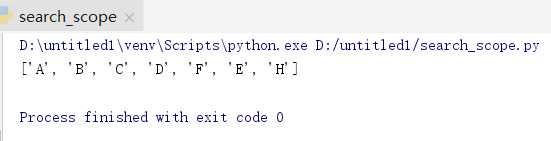图4：遍历次序

代码片段如下：

def search(start,end,grapth):
seach_deque=deque()
seach_deque+=graph[start]
searched=[start]
while seach_deque:
person=seach_deque.popleft()
if node  not in searched:
if node==end:#start<>end
searched.append(end)
return searched
else:
seach_deque+=graph[node]
searched.append(node)
return False

我们再编写一个函数，用来打印出遍历路径节点 ，从BFS遍历路径中找出最短路径，方法： 从循环从路径中遍历，直到找出哪个节点包含了终点，然后向上再遍历哪个父节点包含了该节点，然后倒序输出。

def print_bfs_path(start,end,lst,grapth):
path=[start]
pointer=end
while pointer!=start:
for node in lst:
if pointer in grapth[node]:
pointer=node
path.append(node)
break
print(path[::-1])#倒序打印出来节点列表


本例最终代码调用顺序及输出的最短路径，见下图：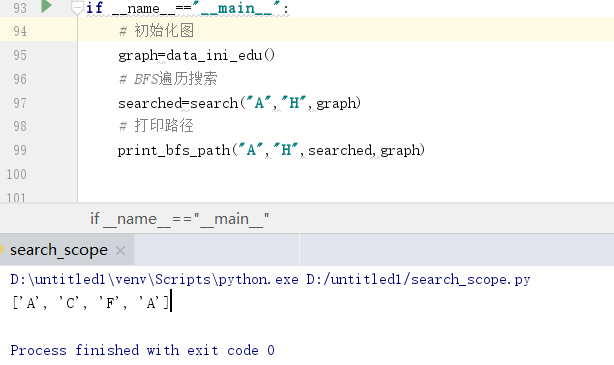总结：1.学习算法一定要了解算法的实际应用场景，是解决什么问题的；2.广度优先搜索是基于图，所以首先要了解图的相关概念，分类；3.第一阶段是看别人代码，第二阶段自己写代码，第三阶段举一反三，模拟一个实际应用进行编码实现，最终才能使用算法。

====================  =================完=======================================================


展开全文最短路径
• from collections import deque graph = {} graph["you"] = ["alice", "bob", "claire"] graph["bob"] = ["anuj", "peggy"] graph["alice"] = ["peggy"] graph["claire"] = ["thom", "jonny"] ...
from collections import deque

graph = {}
graph["you"] = ["alice", "bob", "claire"]
graph["bob"] = ["anuj", "peggy"]
graph["alice"] = ["peggy"]
graph["claire"] = ["thom", "jonny"]
graph["anuj"] = []
graph["peggy"] = []
graph["thom"] = []
graph["jonny"] = []

#起始顶点和结束顶点
start='you'
end='thom'
distance=0
#创建队列
search_queue = deque()
#往队列中加入起始顶点关联的下一批
search_queue+=graph[start]
#print search_queue
#标记已经遍历过的顶点
searched=[start]

#当队列不为空时
while search_queue:
#将队列第一位取出
person = search_queue.popleft()
#print person
#如果该顶点还没有被遍历
if person not in searched:
#且该顶点已经到达目的
if person == end:
searched.append(end)
print searched
#该顶点已经被遍历
else:
# 将此人关联的其他人加入队列
search_queue += graph[person]
searched.append(person)
#print search_queue

输出
['you', 'alice', 'bob', 'claire', 'peggy', 'anuj', 'thom']

但是这个只能得到顶点的遍历顺序(输出searched数组即可)。如果我们想将start=‘you’，
end='thom’这两个顶点间的路径和路径长度求出来，单单上面的代码是不够的。
#打印最短路径
l=[]
endd='thom'
l=[endd]
#没有找到其实顶点you
while endd != 'you':
#遍历searched的顶点
for i in searched:
#如果找到endd的父亲
if endd in graph[i]:
#将父亲加入到数组
l.append(i)
#令endd=父亲顶点（继续找父亲的父亲，直到找到起始顶点）
endd=i
break
print l
print l[::-1]


输出
['thom', 'claire', 'you']
['you', 'claire', 'thom']



展开全文• 这种问题可以用广度优先搜索解决。 首先，数字分布最多会有987654321=362880种，我们建立一个9*362880的二维列表用于存放代表九宫格数字分布的数组。然后往各个方向移动空格，建立另外一个列表用于存放移动到某个...
问题
在3*3的空格中，分别放置1~9的数字，然后将其中某一个置0作为空格，打乱顺序，需求最少步数回恢复到原来的样子。
思路
这种问题可以用广度优先搜索解决。
首先，数字分布最多会有987654321=362880种，我们建立一个9*362880的二维列表用于存放代表九宫格数字分布的数组。然后往各个方向移动空格，建立另外一个列表用于存放移动到某个数字分布时的次数，直到恢复到原来的样子为止。
看网上其他的代码都只是计算出最少次数而已，并没有把每一步如何移动显示出来，这里我用了一个列表作为前驱记录这一步是由哪一步移动得到的，最后只要带入进去就可以得到了。如果有其它的方法欢迎交流。
代码
这里我用python写的，这里li0是打乱了的九宫格，li_goal是要恢复成的样子。
def bool_rules(first,next):
"""判断移动是否合法1-9"""
if (first>9)|(first<1)|(next>9)|(next<1):
return 0
elif (first%3==0)&(next%3==1):
return 0
elif (first%3==1)&(next%3==0):
return 0
elif ((abs(first-next)==3)|(abs(first-next)==1)):
return 1
else:
return 0

def turn(x,y,li):
#1-9
#交换位置，返回数组和空格的位置
temp = li[:]
temp[x-1] = li[y-1]
temp[y-1] = li[x-1]
return temp

def insert_exist(s):
v = 0
for i in range(0,9):
v = v*10 + st[s][i]
if vis.count(v)>0:
return 0
else:
vis.append(v)
return 1

def cmp(q,p):#不同为1 ，相同为0
if len(q)-len(p)!=0:
return 1
x=0
for i in range(0,len(q)):
if q[i]!=p[i]:
return 1
return 0

def run():
st=li0[:]
front = 1
rear = 2
while front<rear:
if cmp(st[front],li_goal)==0:
return front
x=st[front].index(0)+1
for i in move:
if bool_rules(x, x + i):
st[rear]=turn(x,x+i,st[front][:])[:]
if insert_exist(rear):
dist[rear] = dist[front]+ 1
before[rear]=front
rear +=1
front+=1
return -1

if __name__ == '__main__':
li_goal = list(range(1, 10))
global move  # 上下左右
move = [-3, 3, -1, 1]
max = 362880  # 9*8*7*6*5*4*3*2*1
st = [[0 for i in range(9)] for i in range(max)]
vis = 
dist = [0 for i in range(max)]
before = [0 for i in range(max)]
li0=[1,2,3,0,6,9,4,7,8]
li_goal[5-1]=0

a=run()
num=dist[a]
print('次数:',num)
step = [[0 for i in range(9)] for i in range(num+1)]
step[num]=li_goal[:]
for i in range(1,num+1):
b=before[a]
step[num - i]=st[b][:]
a=b
for i in range(0,num+1):
print('step ',i)
print(step[i][0:3])
print(step[i][3:6])
print(step[i][6:9])


结果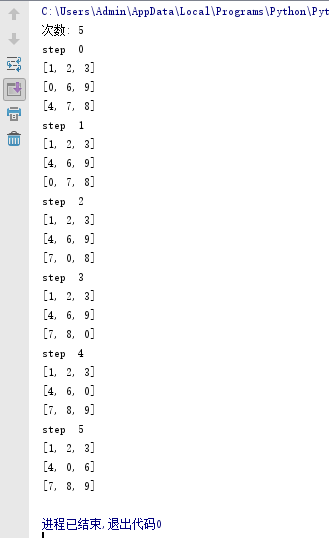展开全文• 要实现的是输入一张 图，起点，终点，输出起点和终点之间的最短路径思路广度优先搜索是以层为顺序,将某一层上的所有节点都搜索到了之后才向下一层搜索； 比如下图： 从0结点开始搜索的话，顺序就是 0， 1，2，4， ...算法
• mapmodel=[ [0,1,1,-1,1], [1,0,-1,1,-1], [1,-1,0,-1,1], [-1,1,-1,0,-1], [1,-1,1,-1,0] ] flag=[1,0,0,0,0] def dfs(current,sumpoint): if sumpoint==5: ... if mapmodel[current][i]==1 and flag[i]==0:...遍历图中的点
• 该问题受《算法图解》第6章寻找朋友圈中的芒果销售商启发，但是在它的基础上做了一些优化，使用了一些很棒的工具以及巧妙的方法（参考classic computer science problems in Python）。下面请看具体描述： 如果我们...namedtuple bfs deque
• 主要介绍了python实现图的深度优先搜索和广度优先搜索相关知识点，对此有兴趣的朋友学习下。
• 主要介绍了python实现广度优先搜索过程解析,文中通过示例代码介绍的非常详细，对大家的学习或者工作具有一定的参考学习价值,需要的朋友可以参考下
• 利用Python实现广度优先搜索BFS 广度优先搜索和深度优先搜索一样，都是最基础的搜索算法，为了体验广度优先搜索BFS的特点，在这篇文章中，我将使用Python来实现一个BFS解答题目的过程。 首先，让我们来看看BFS的定义...队列 算法 数据结构
• 主要介绍了python 递归深度优先搜索与广度优先搜索算法模拟实现 ,非常不错，具有一定的参考借鉴价值，需要的朋友可以参考下
• 广度优先搜索广度优先搜索是一种图算法，主要解决两种问题： 1.从节点A出发，有前往节点B的路径吗？ 2.从节点A出发，前往节点B的哪条路径最短？芒果销售商问题假设你经营着一个芒果农场，需要寻找芒果销售商，以便将......

# python广度优先搜索python 订阅